Mobile QR Code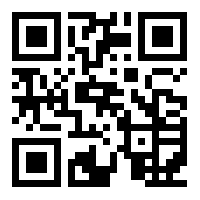1. (School of Physical Education, University of Sanya, Sanya, 572000, China)

Action recognition, Convolutional neural network, Visual relation, Self-attention mechanism

## 1. Introduction

The number of videos onthe Internet has exploded, and the level of computer hardware and information science has significantly improved. However, there are still problems that need to be solved to find out how to accurately obtain valuable information from action images from online physical education videos and perform intelligent identification and classification. A convolutional neural network (CNN) is mainly used in deep learning in computer vision tasks. The convolution operation is used to extract the features of an input image, and the underlying features of the data are combined to form discriminative high-level features . In addition, CNNs can also learn and classify features from massive data, showing very good model generalization ability .

<New paragraph> Skeleton point modality has attracted the attention of many researchers . Action image recognition using skeleton point modality can greatly reduce the adverse effects caused by a lens and background noise, so this method is more suitable for recognition of action images in medium <note: ambiguous> . In order to optimize the action recognition method using a bone point pattern, a post fusion method of spatio-temporal depth features based on a CNNwasstudied, and the bone point pattern was improved by using a spatio-temporal graph attention network (STGAT). The proposed CSTGAT model can identify human action images in video clips, provide a new way of teaching physical education, assist in distance learning, and promote the harmonious and stable development of society <note: ambiguous/awkward>.

We used a CNN to take joints as nodes of graph network and used a fixed adjacency matrix to describe the relationship between nodes, which can quickly update and obtain the characteristics of other nodes. STGAT was used to capture the cross spatio-temporal information in the spatio-temporal neighborhood, expand the spatial receptive field of nodes, and introduce a separation learning strategy to accurately aggregate the features of each order of the spatio-temporal neighborhood. A dynamic time weighting strategy can dynamically weigh the information of each frame in the local space-time neighborhood. A display motion capture strategy reduces the redundancy of local spatiotemporal features and enhances the accuracy of recognition.

## 2. Related Work

As a tool to solve the problems existing in the field of understanding video and computer vision in recent years, action recognition technology has attracted widespread public attention. Action image recognition requires judging and classifying the actions existing in multiple frames of images in a video clip, and attaching corresponding labels . Many scholars have conducted in-depth discussions on this issue. Anithaet al. set up a robust human action recognition system based on image processing and used it to detect human behavior representations . Nwoye et al. designed a novel spatial attention to capture single action triples in the scene (i.e., a class activation guided attention mechanism) and analyzed surgical actions in endoscopic videos to achieve accurate action recognition . Jiang et al. established an artificial deep learning framework based on the SMO algorithm optimization model and artificial intelligence-based motion combination training action recognition model. They studied methods to improve the accuracy of motion combination action recognition .

<New paragraph> Based on the trampoline motion decomposition method for deep learning image recognition, Liu et al. explored the key steps of an athlete's trampoline somersault . Silva et al. developed a skeleton-driven action recognition approach based on spatiotemporal representations of images and CNNs to explore stereotyped movements in children with autism spectrum disorder . Ali et al. explored the visible spectrum of video media for action recognition and used Beta-Liouville Hidden Markov Models for a multimodal action recognition . Kim et al. studied multi-view action recognition and classification using skeleton-based features for viewpoint-aware action recognition .

With the development of deep learning technology, the use of deep CNNs to classify action images has attracted the attention of many researchers. The structure of a CNNis becoming increasingly simpler, and the performance and generalization ability of the model are stronger than in other classification methods, so it has been widely used in various fields . Zhou et al. designed a short text classification algorithm based on semantic expansion and a CNN to extract effective information from a large number of original texts and improve the classification performance for short texts. A test with four datasets showed that the proposed model hada better effect than the most advanced models, and the computational difficulty was lower .

<New paragraph> Satyanarayanaet al. built a CNN model to detect and classify vehicles on a road in the construction of intelligent transportation. This model does not require real-time implementation, which is more convenient, and its detection accuracy is as high as 98.5% . Eldho et al. effectively removed the Gaussian impulse noise in digital images using a new type of pseudo-CNN without adjustable parameters for image preprocessing and then used a CNN optimization model to process images. The results showed that this method had better qualitative and quantitative results than the best technology at present and can also remove noise efficiently .

<New paragraph> Hu et al. built a network integration framework based on a CNN to enhance local and regional motion history images in order to solve the problem of facial expression in video sequences . Jagannathan et al. used a CNN prediction model to make timely predictions of land and natural resources information for mitigating the urban heat island phenomenon . Focusing on slow retrieval speed and easy loss of information in video retrieval, Chen et al. used 3D-CNN technology to extract spatiotemporal image features and conducted experiments based on a large number of datasets. It has the advantage of high efficiency and can effectively improve the retrieval speed of video images .

Comprehensively, it can be observed that relevant domestic and foreign researchin the field of action image recognition and CNNs has achieved good evaluations in practice. Therefore, we used aCNN to optimize the action recognition method, design a spatiotemporal attention network based on the self-attention mechanism, improve skeleton point modality, construct an action recognition model for video clips, and realize a new teaching mode to meet needs for physical exercise and learning.

## 3. Construction of CSTGAT Model based on CNN

### 3.1 Spatiotemporal Depth Feature Fusion based on CNN

Sports actions in action image recognition are special and complex and cannot be accurately identified. More attention should be paid to the processing of actions of various parts of the human body. The actions of the human body are discriminative in not only the spatial dimension, but also in the time dimension . When performing a recognition task with human action images, it is necessary to deeply mine the features in the spatiotemporal dimension from online videos. In the aspect of extracting spatial depth features, a method of combining a depth map and RGB map is adopted. While extracting relevant features, it can also accurately distinguish the scene level and human body in the image.

<New paragraph> A CNN can perform deep learning from a large number of samples, thereby obtaining corresponding features and optimizing the long-term and complex feature extraction process. Moreover, it can directly process the collected two-dimensional action images, which has strong applicability. Its structure is used in depth maps and RGB maps. The difference between the two types of graphs is due to the difference in input signals, so distinctive features are mined. The underlying features of the CNN focus on mining common features, and the high-level features are biased towards extracting unique features of the image.

<New paragraph> Let the graph structure be $Q=\left(R,L\right)$, $R=\left(r_{1},r_{2},\cdots ,r_{S}\right)$ be the $S$ graph nodes of joints, $L$be the graph edge of bones between joints, and the $S\times S$ adjacency matrix $O$ be the connection between joints. If $r_{i}$ is connected with $r_{j}\,,$ $O_{ij}=1;$ otherwise, $O_{ij}=0$. In general, $Q$ is an undirected graph, so $O$ is a symmetric matrix. Given the input vector $U$and graph structure, the graph convolution operation of each time step can be calculated, as shown in Eq. (1).

##### (1)
$U^{out}=\theta \left(YU^{in}\chi ^{-\frac{1}{2}}\left(O+I\right)\chi ^{-\frac{1}{2}}\right)$

where $U^{in}$ is the input feature, $U^{out}$ is the output vector, $Y$ is a feature transformation matrix that can be trained, $\chi$ is an angle matrix normalized to $O$, and $I$ is $O$ to increase self-loop connection to maintain its own characteristics. <note: ambiguous> We used the Softmax loss function. $Z$represents the output of the last layer of the neural network, which is basically a vector of dimension <note: ambiguous>. The definition of the Softmax loss function is expressed as Eq. (2).

##### (2)
$\sigma \left(x_{a}\right)=\exp \frac{1}{\sum _{a=1}^{Z}\exp \left(x_{a}\right)}$

In order to reduce the error generated by the loss function, the parameters of the CNN are optimized by using the stochastic gradient descent algorithm, and the iterative process is stopped when the network converges to a stable trend. We input the depth map and the independent RGB image into the deep learning CNN model for feature extraction and then fuse the extracted results into new features. The new features can have the spatial information of both the RGB image and the individual depth image. The obtained new feature is also the spatial depth feature (SDF), which is calculated with Eq. (3).

##### (3)
$SDF=\frac{A_{1}}{A_{1}+A_{2}}SDF_{1}+\frac{A_{2}}{A_{1}+A_{2}}SDF_{2}$

where $A_{1}$ represents the accuracy of RGB map calculation, $A_{2}$ represents the accuracy of depth map calculation, $SDF_{1}$ is the feature of the RGB map, and $SDF_{2}$ is the feature of the depth map.

Human movements in teaching videos contain not only spatial characteristics, but also temporal characteristics, so it is also necessary to extract the temporal depth characteristics of action images. A commonly used deep learning method for processing temporal feature information is based on the two-layer structure of a recurrent neural network (RNN), in which the calculation of the output layer is shown in Eq. (4).

##### (4)
$h_{t}=f\left(h_{t-1},u_{t}\right)$

where $f$represents the model function that the RNN needs to train, and $h_{t}$represents the output layer. The RNN is iteratively processed through the time scale of the sequence. Therefore, the RNN has excellent application effects in modeling and feature extraction of sequence data.

The dimension of temporal depth feature extraction is calculated using the cross-entropy loss function, which is defined in Eq. (5).

##### (5)
$L\left(v_{t},v'_{t}\right)=-\sum _{t}v_{t}\log v'_{t}$

where $v_{t}$ represents the correct label at the time point $t$, and $v'_{t}$ represents the predicted label calculated by the network. In order to control the calculation result of the loss function in a lower region, the gradient optimization parameters of the loss function $B$ are calculated, and the calculation of the total gradient is shown in Eq. (6).

##### (6)
$\frac{\partial L}{\partial B}=\sum _{t}\frac{\partial L}{\partial B}$

According to the calculated loss and gradient results, the weights can be automatically adjusted, and finally, the optimized network model can be obtained by learning and training. A traditional RNN has a problem of gradient dispersion. When the information flow of the teaching video is too long, the number of iterations is so large that the gradient explosion makes it difficult to carry out the training task. In order to solve the problem of gradient dispersion, the LSTM-RNN method was used to learn temporal depth features. The unit structure of LSTM is shown in Fig. 1.

There is a state C in the internal unit structure of LSTM, which can be iteratively updated as the time point of the input sequence increases, which solves the problem of gradient dispersion. The late fusion method was adopted for the feature fusion of spatial depth features and temporal depth features. First, the probabilities of spatial and temporal depth features need to be superimposed using linear weighting before being output to the subsequent process, and then the predicted value is obtained. The calculation of late fusion is shown in Eq. (7).

##### (7)
$P=\frac{1}{N}\left(\sum _{b=1}^{N}\varepsilon P_{1}+\left(1-\varepsilon \right)P_{{_{2}}}\right)$

where $\varepsilon$ represents the weighting parameter, $P$ represents the final prediction probability, $N$ is the number of sample features after multiple calculations and analysis of the video, $P_{1}$ represents the output probability of spatial depth features, and $P_{2}$ represents the output probability of temporal depth features.

##### Fig. 1. Unit structure of LSTM.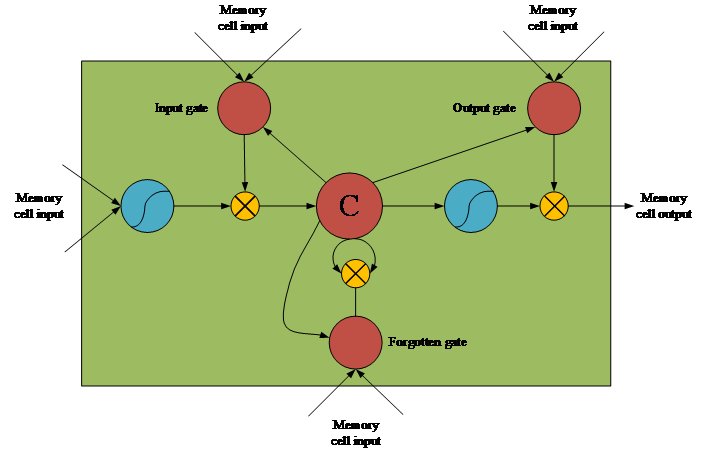### 3.2 CSTGAT Model based on Skeleton Point Action Recognition

Due to the rapid development of wearable motion capture devices and human motion estimation algorithms, a method of motion recognition through skeleton points is more and more widely used. Due to the collected skeleton point data, the influence of lens movement, light transformation, and image noise can be largely avoided, and the method of using skeleton point data for action recognition pays more attention to the movements of the human body. The method of using high-order adjacency matrix decomposition has disadvantages of high computation cost and inability to distinguish the importance of neighbors. Therefore, STGAT based on a self-attention mechanism is introduced to solve the problem. STGAT can perform adaptive computational tasks on the connections between the physical structure of human actions in a local spatiotemporal neighborhood. The self-attention operator in each time step is defined as Eq. (8).

##### (8)
$D_{e}=\frac{1}{C\left(U_{e}^{in}\right)}\sum _{\forall i}f\left(U_{e}^{in},U_{i}^{in}\right)g\left(U_{i}^{in}\right)$

where $D_{e}$ represents the weight of the connection between the node $e$ and other nodes, $v_{e}$ represents the index of the output layer, and $i$ represents the index of all possible node positions. The function $C$ normalizes the obtained results, the function $f$ represents the connection weight between two nodes $v_{e}$ and $v_{i}$, and $g$ is used to carry out the operation of transforming the dimension of the features ($g=1$).

According to the adjacency matrix $D,$ the output features $U^{out}$ can be calculated with Eq. (9).

##### (9)
$U^{out}=\vartheta \left(EU^{in}D\right)$

where $\vartheta$ is the activation function, and $E$ represents a feature transformation matrix that can be learned. The study uses an embedded Gaussian function to measure the similarity of a set of vectors, and its definition is expressed as Eq. (10).

##### (10)
$s\left(u_{e},u_{i}\right)=e^{\xi {\left(u_{e}\right)^{T}}\tau \left(u_{i}\right)}$

Eq. (10) is a function that maps the $\xi$ feature $u_{e}$ to high-dimensional space, which is the function that maps the $\tau$ feature $u_{i}$ to the high-dimensional space. The embedded Gaussian function can be highly adapted to the Softmax function. Through the determined position $e$, the normalization factor $C$ can be used to implement the subsequent operations of $\frac{1}{C\left(u\right)}e^{\xi {\left(u_{e}\right)^{T}}\tau \left(u_{i}\right)}$ in the form of Softmax along the dimension $i$. Through this equation, the self-attention module can be planned. Weinstantiate $\xi$ and $\tau$ in a 1${\times}$1 convolution, and the output channel can be set to $C_{e}<C$ to reduce the computational consumption. When calculating the result of the output channel, $C_{out}/d$ is used to regulate the amount of calculation of the output channel. The process of obtaining the self-attention module is shown in Fig. 2.

The setting of the multi-head attention module can be used to learn different types of adjacency matrices, which represent the different connection relationships between nodes. By parallelizing the independent self-attention modules $K$, learning types of adjacencies with inconsistent matrix structure. <note: ambiguous (incomplete sentence> The calculation of the output channel is expressed as Eq. (11).

##### (11)
$U^{out}=\vartheta \left(\frac{1}{K}\sum _{k=0}^{K}E_{k}U^{in}D_{k}\right)$

where $D_{k}$ represents the adjacency matrix calculated by the $k$th self-attention module, and $E_{k}$ represents the feature transformation matrix calculated by the $k$th self-attention module.

<New paragraph> The parallel processing of the self-attention mechanism provides a more flexible and stable solution for establishing different kinds of connections between skeletal joints. In order to make the information of each convolution module reach the target node through a shorter path and remove the background noise more effectively, the scope of the spatial graph attention network is expanded to the time domain, and then the effective information in the spatiotemporal neighborhood can be captured. The sampling operation is performed by using a sliding window with range $\gamma$ and expansion coefficient $m$. The time steps of the input sequence are sampled to generate the corresponding local action sequence expressed as Eq. (11).

##### (12)
$U_{\gamma }^{t}=\left\{u_{t-\gamma /2;t+\gamma /2}\in R^{C\times tW}\left| t\in Z,0\leq t\leq T\right.\right\}$

where $\gamma$ is used to control the time range of the sampling sequence, and $m$ represents the selection of a frame from a video segment. The spatiotemporal attention network calculates each frame of images selected to obtain the corresponding spatiotemporal adjacency matrix, which is defined in Eq. (13).

##### (13)
$D_{\gamma }^{t}=\left[D_{1}^{t},D_{2}^{t},\ldots ,D_{W}^{t}\right]$

The spatiotemporal adjacency matrix can be obtained by calculating all the neighbors of the local spatiotemporal neighborhood and the similarity of the point $D_{\gamma }^{t}$. The spatiotemporal network calculates the output vector of each frame image according to Eq. (14).

##### (14)
$\left[U^{out}\right]^{t}=\psi \left(E\left[U_{\gamma }^{in}\right]^{t}D_{\gamma }^{t}\right)$

In order to achieve the research goals, it is necessary to expand the scope of STGAT through a method of separation learning and divide the joints in the local spatiotemporal neighborhood. By grouping, STGAT only needs to calculate the connection weights of each edge in each group, and the extracted features are connected to obtain all multi-scale features. Then, two methods are introduced to dynamically weigh STGAT. An optimization parameter that can be updated with the network is added:$F_{DTW}$. The adaptive dynamic time weighting process is shown in Fig. 3.

The adaptive dynamic weighting method can only dynamically weigh the action images in the local spatiotemporal neighborhood, so an explicit motion capture method is needed to remove the excessively extracted features in the local spatiotemporal neighborhood and increase the time perception for each frame of the action image. The explicit motion capture strategy not only highlights the changes of human motion, but also cooperates with the adaptive dynamic temporal weighting method to effectively reduce the redundant features extracted. Through fusion of spatiotemporal depth features based on a CNN and the use of skeleton points for human action recognition, a skeleton point self-attention mechanism action recognition model based on a CNN called theCSTGAT model was constructed. The specific flow of the CSTGAT model is shown in Fig. 4.

Three evaluation indicators were used to evaluate the quality of the prediction model: accuracy, recall, and the F1 value. First, the definition of accuracy is expressed as Eq. (15):

##### (15)
$Accuracy=\frac{TP}{TP+NP}$

where $TP$represents the number of positive data with prediction results that are consistent with the actual situation, and $NP$represents the number of positive data withprediction results that are inconsistent with the actual situation. The recall rate is calculated with Eq. (16):

##### (16)
$\mathrm{Re}\text{call}=1-\frac{TP}{P}$

where $P$ represents the total number of positive samples. The F1 value can be obtained by calculating the harmonic mean of precision and recall. The larger the value is, the better the prediction effect of the model will be. The F1 value is calculated with Eq. (17):

##### (17)
$F_{1}=\frac{2\ast accuracy\ast \text{recall}}{accuracy+\text{recall}}$
##### Fig. 2. The flow of the self-attention module.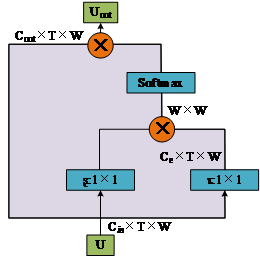##### Fig. 3. Adaptive dynamic time weighting process.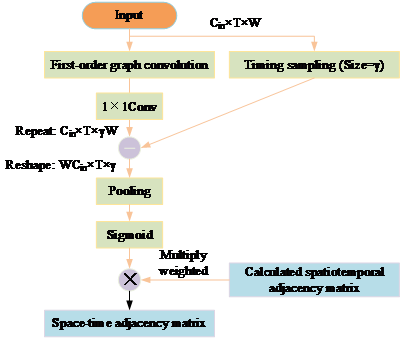##### Fig. 4. The flow of the CSTGAT model.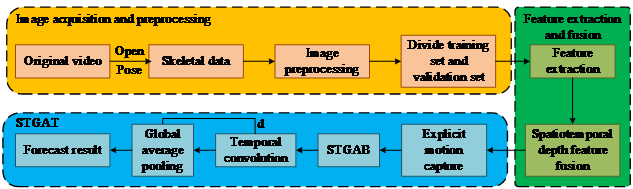## 4. Performance Analysis of CSTGAT Model based on CNN

In order to verify the relevant performance of the CSTGAT model based on a CNN, three action recognition databases were selected for an experiment: MSR 3D Online Action, NTU RGB+D 60, and NTU RGB+D 120. There are 49,286 video actions in the MSR 3D Online Action dataset, which are divided into 60 categories. There are about 350 images in each video. There are 5776 video actions in the NTU RGB+D 60 dataset, which are also divided into 60 categories. There are 91,854 video actions in the NTU RGB+D 120 dataset, which are divided into 120 categories.

<New paragraph> At present, the most mainstream video action recognition models mainly include the TSN model and SOTA model. The TSN model can sample a series of short clips from a bottle <note: ambiguous> and obtain video prediction results based on the consensus of these clips, which can be very useful for managing the classification of long videos. The SOTA model has a fast reasoning speed and high accuracy . Therefore, the TSN model, SOTA model, and CSTGAT model were selected for comparison. The data samples were divided into a training set and verification set according to different shooting angles. In the process of CNN training, the punitive loss function model and the cross-entropy loss function model were calculated, and training results using two different loss function models were obtained, as shown in Fig. 5.

It can be seen in Fig. 5(a) that the loss function uses a model with a penalty term with an average accuracy of 40.7%. Moreover, the value of the cost function fluctuates greatly, especially during the training process of the CNN. For the loss function model of the penalty term, it is difficult to achieve excellent convergence. In Fig. 5(b), the loss function using the model with cross entropy has an average accuracy of 91.6%, and the value of the cost function is low and stable, so the model can have excellent convergence. The experimental results show that the loss function model using cross entropy can make the model reach a stable target convergence state more quickly and effectively, so it is beneficial to study the loss function using cross entropy. After the training of the CNN, the convergence effect of different models can be obtained, as shown in Fig. 6.

As shown in Fig. 6, the convergence state of the CSTGAT model is better compared to the other two action recognition models. To achieve stable convergence, the CSTGAT model needs only 217 iterations, the SOTA model needs 262 iterations, and the TSN model needs 285 iterations. The experimental results show that the convergence performance of the CSTGAT model is better, and the network training is completed well. In the experiment, different feature methods were used for training and verification in two datasets, and the accuracy results obtained are shown in Table 1.

It can be observed from Table 1 that in the NTU RGB+D 120 dataset, the training accuracy of the CSTGAT model is 97.2%, and the verification accuracy is 97.5%. The training accuracy of the TSN model is 88.6%, and the validation accuracy is 88.2%. The training accuracy of the SOTA model is 90.5%, and the verification accuracy is 90.2%. The validation accuracy of CSTGAT model is higher thanthose of the TSN model and SOTA model by 9.3% and 7.3%,respectively.

<New paragraph> In the MSR 3D Online Action dataset, the training accuracy of the CSTGAT model is 96.1%, and the verification accuracy is 96.9%. The training accuracy of the SOTA model is 89.6%, and the verification accuracy is 90.1%. The training accuracy of the TSN modelis 86.5%, and the validation accuracy is 87.8%. Compared with the CSTGAT model, TSN model’svalidation accuracy is 8.8% lower, and SOTA model’s validation accuracy is 6.8% lower.

<New paragraph> In the NTU RGB+D60 dataset, the training accuracy of the CSTGAT model is 97.2%, and the verification accuracy is 96.8%. The training accuracy of the TSN model is 86.5%, and the validation accuracy is 87.8%. The training accuracy of the SOTA model is 90.9%, and the verification accuracy is 91.5%. The validation accuracy of CSTGAT model is higher than the TSN model and SOTA model’s accuracy by 9.0% and 5.3%, respectively. In the experiment, different models were used for calculation in the verification set, and the comparison results between the predicted value and the actual value of different action recognition models were obtained, as shown in Fig. 7.

Fig. 7 shows that the accuracy of the SOTA model is 91.50%, the accuracy of the CSTGAT model is 98.47% , and the accuracy of the TSN model is 69.15%. Compared with the accuracy of the SOTA model, the accuracy of the CSTGAT model is 6.97%higher. Compared with the TSN model, the accuracy of the CSTGAT model is 29.32% higher. The results show that the CSTGAT model can handle a large amount of calculation while having high accuracy. In the experiment, different models were used for 100 calculations in the validation set, and the obtained precision and recall results are shown in Fig. 8.

As shown in Fig. 8, the precision and recall curves of the CSTGAT model are stable, and with an increase of the number of experiments, the average precision of the CSTGAT model is 97.43%. The rate curve fluctuates greatly, and the average accuracy of the TSN model is 86.59%, while the average accuracy of the SOTA model is 90.71%. The precision and recall rates of the CSTGAT model are higher than those of the other two action recognition models, indicating that the CSTGAT model has higher accuracy.

<New paragraph> The average recall rate of the CSTGAT model is 71.65%, while that of the SOTA model is 61.86%. The average recall rate of the TSN model is 49.53%, which is 22.03% lower than that of the CSTGAT model. The results show that the CSTGAT model has higher accuracy and a more comprehensive query rate. The three action recognition models were tested on the validation set, and the performance of the models was evaluated using the F1 value. The variation of the F1 value of the three action recognition models is shown in Fig. 9.

It can be observed from Fig. 9 that after 100 tests, the CSTGAT model has a more stable F1 curve. The experimental results show that the average F1 value of the CSTGAT model is 96.83%, while that of the SOTA model is 85.94%.The average F1 value of the TSN model is 69.11%, which is lower than that of the CSTGAT modelby 27.72%. With the increase of the number of iterations, the CSTGAT model is very stable with little fluctuation. The SOTA model and TSN model have greater fluctuationrange and frequency. The SOTA model has the largest fluctuation range and the worst model expressiveness. Based on the results, the CSTGAT action recognition model can achieve extremely high accuracy and precision and can accurately identify human movements in videos, which is conducive to the development of online teaching methods.

##### Fig. 5. Changes in the training process of CNNs for models using different loss functions.##### Fig. 6. Convergence process of different models in CNN training.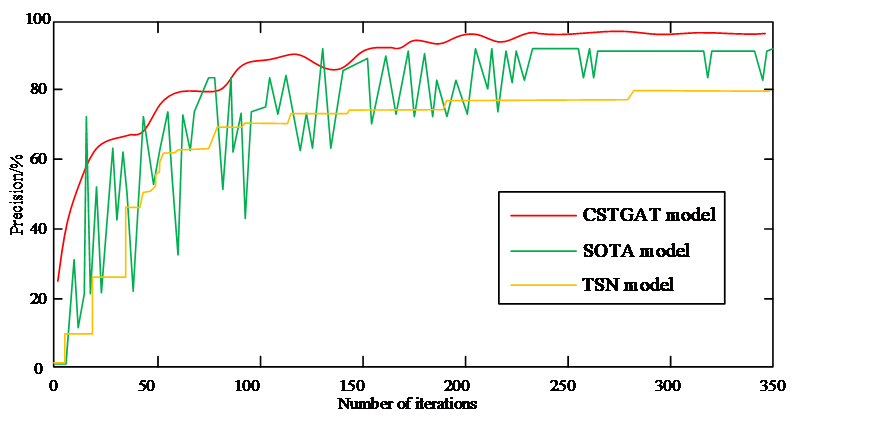##### Fig. 7. Error analysis of different models.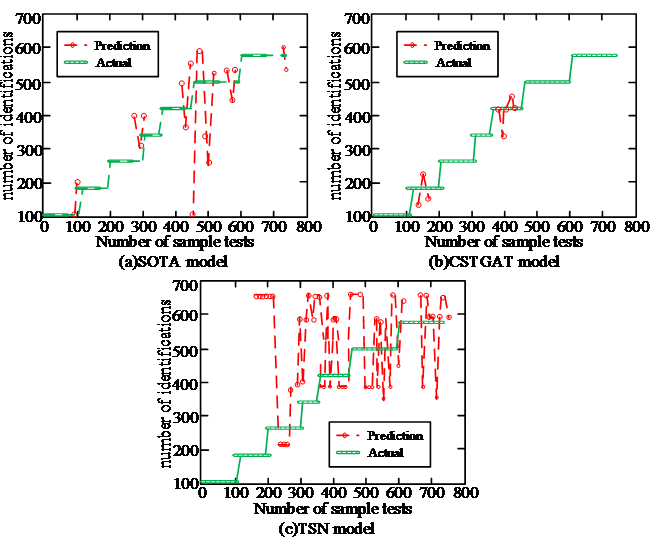##### Fig. 8. Precision and recall for different models.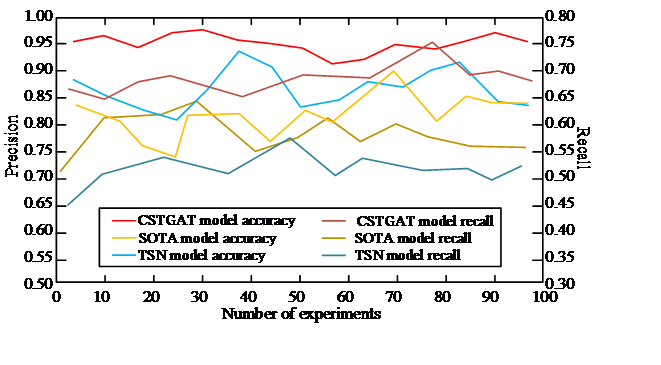##### Fig. 9. Variation of the F1 value for different models.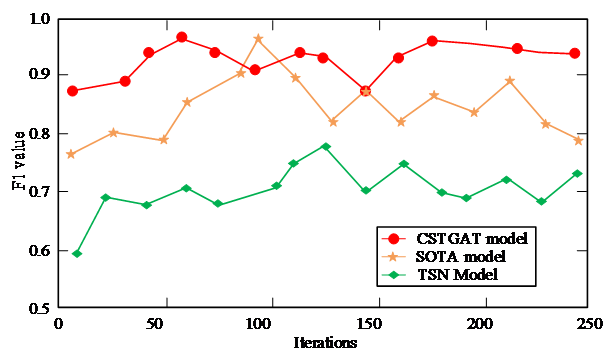##### Table 1. Comparison of the accuracy of CSTGAT model and other latest models on three datasets.
 Dataset Model Accuracy/% Training set Validation set NTU RGB+D 120 TSN 88.6 88.2 SOTA 90.5 90.2 CSTGAT 97.2 97.5 MSR 3D Online Action TSN 87.9 88.1 SOTA 89.6 90.1 CSTGAT 96.1 96.9 NTU RGB+D 60 TSN 86.5 87.8 SOTA 90.9 91.5 CSTGAT 97.2 96.8

## 5. Conclusion

This study provided a solution for late fusion of spatiotemporal depth features based on CNNs and skeleton point actions based on a self-attention mechanism. Combining the recognition methods, a skeleton point action recognition model based on a CNNwas constructed. The results showed that after the training of the CNN, the CSTGAT model achieved stable convergence within only 217 iterations. In contrast, the SOTA model needed45 more iterations than the CSTGAT model, andthe TSN model needed 68 more iterations. The accuracy of the CSTGAT model was 98.47%, which is 10.84% higher than that of the SD-Net model and 29.32% higher than that of the TSN model.

<New paragraph> The accuracy of the CSTGAT model was 97.43%, whichwas 10.84% higher than that of the TSN model and 6.72% higher than that of the SOTA model. The recall rate of the CSTGAT model was 71.65%, which was9.79% lower than that of the SOTA model and 22.03% lower than that of the TSN model. After 100 tests, the F1 value of the CSTGAT model was 96.83%, whichwas 10.89% higher than that of the SOTA model and 27.72% higher than that of the TSN model.

<New paragraph> In summary, the CSTGAT model can realize action recognition more efficiently and accurately and has better model expressiveness. However, there are still shortcomings in this research. The parameters of the model are too large, and the structure of the model needs to be simplified in future research.

### REFERENCES

1
Guo. M, Yu. Z, Xu. Y, Li. C. “ME-Net: A deep convolutional neural network for extracting mangrove using sentinel-2A data,” Remote Sensing, vol. 13, no. 7, pp. 1-24, 2021.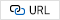2
Wu. H, Zhou. B, Zhu. K, Shang. C, Tam. HY, Lu. C. “Pattern recognition in distributed fiber-optic acoustic sensor using intensity and phase stacked convolutional neural network with data augmentation,” Optics Express, vol. 29, no. 3, pp. 3269-3283, 2021.3
Rastgoo. R, Kiani. K, Escalera. S. “Hand sign language recognition using multi-view hand skeleton,” Expert Systems with Applications, vol. 150, no. 8, pp. 113336, 2020.4
Tsai. MF, Chen. CH. “Spatial temporal variation graph convolutional networks (STV-GCN) for skeleton-based emotional action recognition,” IEEE Access, no. 9, pp. 13870-13877, 2021.5
Gao. P, Zhao. D, Chen. X. “Multi-dimensional data modelling of video image action recognition and motion capture in deep learning framework,” IET Image Processing, vol. 14, no. 7, pp. 1257-1264, 2020.6
Anitha. U, Narmadha. R, Sumanth. DR, Kumar. DN. “Robust human action recognition system via image processing,” Procedia Computer Science, no. 167, pp. 870-877, 2020.7
Nwoye. CI, Yu. T, Gonzalez. C, Seeliger. B, Mascagni. P, Mutter. D, Padoy. N. “Rendezvous: Attention mechanisms for the recognition of surgical action triplets in endoscopic videos,” Medical Image Analysis, no. 78, pp. 102433, 2022.8
Jiang. H, Tsai. SB. “An empirical study on sports combination training action recognition based on SMO algorithm optimization model and artificial intelligence,” Mathematical Problems in Engineering, no. 2021, pp. 1-11, 2021.9
Liu. Y, Dong. H, Wang. L. “Trampoline motion decomposition method based on deep learning image recognition,” Scientific Programming, vol. 2021, no. 9, pp. 1-8, 2021.10
Silva. V, Soares. F, Leo. CP, Esteves. JS, Vercelli. G. “Skeleton driven action recognition using an image-based spatial-temporal representation and convolution neural network,” Sensors, vol. 21, no. 13, pp. 4342.11
Ali. S, Bouguila. N. “Multimodal action recognition using variational-based Beta-Liouville hidden Markov models,” IET Image Processing, vol. 14, no. 17, pp. 4785-4794.12
Kim. SH, Cho. D. “Viewpoint-aware action recognition using skeleton-based features from still images,” Electronics, vol. 10, no. 9, pp. 1118, 2021.13
Xuan. P, Gong. Z, Cui. H, Li. B, Zhang. T. “Fully connected autoencoder and convolutional neural network with attention-based method for inferring disease-related lncRNAs,” Briefings in Bioinformatics, no. 3, pp. 89-91, 2022.14
Wang. H, He. J, Zhang. X, Liu. S. "A Short Text Classification Method Based on N-Gram and CNN," Chinese Journal of Electronics, vol.29, no. 2, pp. 248-254, 248-254, March. 2020.15
Abraham. L, Sasikumar. M. “Vehicle Detection and Classification from High Resolution Satellite Images,” Journal of Bacteriology, Vol. 2, no. 1, pp. 1-8, November, 2014.16
Mafi. M, Izquierdo. W, Martin. H, Cabrerizo. M, Adjouadi. M. “Deep convolutional neural network for mixed random impulse and Gaussian noise reduction in digital images,” IET Image Processing, Vol. 14, no. 3, pp. 3791-3801, 2020.17
GS Hayes, SN Mclennan, JD Henry, LH Phillips, I Labuschagne. “Task characteristics influence facial emotion recognition age-effects: A meta-analytic review,” Psychology and Aging, no. 2, pp. 295-315, January 2020.18
Jagannathan. J, Divya. C. “Deep learning for the prediction and classification of land use and land cover changes using deep convolutional neural network,” Ecological Informatics, vol. 65, no. 15, pp. 101412, 2021.19
Chen. H, Hu. C, Lee. F, Lin. C, Yao. W, Chen. L, Chen. Q. “A supervised video hashing method based on a deep 3d convolutional neural network for large-scale video retrieval,” Sensors, vol. 21, no. 9, pp. 3094, 2021.20
Ji. R. “Research on basketball shooting action based on image feature extraction and machine learning,” IEEE Access, no. 8, pp. 138743-138751, 2020.21
Chen. C, Song. J, Peng. C, Wang. G, Fang. Y. “A Novel Video Salient Object Detection Method via Semisupervised Motion Quality Perception,” IEEE, vol. 32, no. 5, pp. 2732-2745, 2019.## Author

Yan Shi, August 20, 1986, female, associate professor, master. She graduated from Xi’an Institute of physical education in July 2008, majoring in human movement science. She graduated from Xi’an Institute of physical education in July 2011, majoring in human movement science. Now she works in Sanya University, school of physica education. She has published 10 academic articles and participated in 4 scientific research projects.